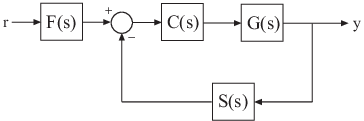## Numeric Model of SISO Feedback Loop

This example shows how to interconnect numeric LTI models representing multiple system components to build a single numeric model of a closed-loop system, using model arithmetic and interconnection commands.

Construct a model of the following single-loop control system.The feedback loop includes a plant G(s), a controller C(s), and a representation of sensor dynamics, S(s). The system also includes a prefilter F(s).

1. Create model objects representing each of the components.

```G = zpk([],[-1,-1],1); C = pid(2,1.3,0.3,0.5); S = tf(5,[1 4]); F = tf(1,[1 1]);```

The plant G is a zero-pole-gain (`zpk`) model with a double pole at s = –1. Model object C is a PID controller. The models F and S are transfer functions.

2. Connect the controller and plant models.

`H = G*C;`

To combine models using the multiplication operator `*`, enter the models in reverse order compared to the block diagram.

Tip

Alternatively, construct H(s) using the `series` command:

`H = series(C,G);`
3. Construct the unfiltered closed-loop response $T\left(s\right)=\frac{H}{1+HS}$.

`T = feedback(H,S);`

Caution

Do not use model arithmetic to construct `T` algebraically:

`T = H/(1+H*S)`

This computation duplicates the poles of `H`, which inflates the model order and might lead to computational inaccuracy.

4. Construct the entire closed-loop system response from r to y.

`T_ry = T*F;`

`T_ry` is a Numeric LTI Model representing the aggregate closed-loop system. `T_ry` does not keep track of the coefficients of the components `G`, `C`, `F`, and `S`.

You can operate on `T_ry` with any Control System Toolbox™ control design or analysis commands.